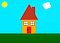# Teach Your Kids to Code by Using the Turtle Librarydraw a house

Coding for kids not only helps them to improve mathematics and problem-solving skills but also encourages them to be more confident in life and eventually in the workforce.

Among all the programming languages, Python is the most suitable one for kids to learn. It is used in college courses and by many tech companies like Google and IBM. However, without graphics, it is not intuitive to understand for a young kid. Fortunately, the Turtle Library is the rescue.

The Turtle Library is a popular way to introduce a young kid to start programming. It was part of the original Logo programming language developed by Wally Feurzeig, Seymour Papert, and Cynthia Solomon in 1967.

Now, let’s first have a look at a few examples.

## Example 1: Draw a square

`from turtle import *home()speed(1)goto(-100,-100)for i in range(4):    forward(200)    left(90)turtle.done()`

## Example 2: Draw a rainbow

`import turtleimport colorsysturtle.speed(0)turtle.hideturtle()turtle.bgcolor('light blue')turtle.title('49-Color Rainbow')turtle.setup(700,700)num_colors = 49radius = 300penwidth = 20*7/num_colorshue = 0for i in range(num_colors):    (r,g,b) = colorsys.hsv_to_rgb(hue,1,1)    draw_one_color_arc(0,-100,radius,penwidth,(r,g,b))    radius -= (penwidth-1) #overlapping a little removes the gaps    hue += 0.9/num_colors`

## Example 4: Draw a Sudoku board

There is a collection of useful commands that is important to create the above artwork and games. So let’s list a few of them.

## Basic Moving Commands

• `turtle.forward(x)`: Moves the turtle forward in the direction it is facing by x steps
• `turtle.back(x)` : Moves the turtle backward from its facing direction by x steps
• `turtle.left(d)`: Turns the turtle degrees counterclockwise
• `turtle.right(d)`: Turns the turtle x degrees clockwise
• `turtle.goto(x,y=None)` : Move the turtle to an absolute position. If the pen is down, draw a line.
• `turtle.circle(radius)` : Draw a circle with a given radius.

## Tell Turtle’s State Commands

• `turtle.position()` | `pos()` : Return the turtle’s current location (x,y)
• `turtle.xcor()` : Return the turtle’s x coordinate.
• `turtle.ycor()` : Return the turtle’s y coordinate.
• `turtle.distance()` : Return the distance from the turtle’s current position to (x,y)

## Pen Control Commands

• `pendown()` : Pull the pen down — drawing when moving.
• `penup()` : Pull the pen up — no drawing when moving.
• `pensize()` : Set the line thickness to width or return it.
• `isdown()` : Return `True` if the pen is down, `False` if it’s up.
• `turtle.pen(pen=None)` : Return or set the pen’s attributes in a “pen-dictionary”, attributes are following
`{  'shown': true,   'pendown': true,   'pencolor': 'black',   'fillcolor': 'black',   'pensize': 3,   'speed': 3,   'resizemode':   'noresize',   'stretchfactor': (1.0, 1.0),   'shearfactor': 0.0,   'outline': 1,  'tilt': 0.0}`

## Initial Turtle State

• Initial position: (0, 0) 
• Initial direction: East (0°) 
• Color: Black 
• Line width: 1 pixel 
• Pen State: down (ready to draw)

Developer in China, AI and machine learning enthusiast

## More from Jerry An

Developer in China, AI and machine learning enthusiast